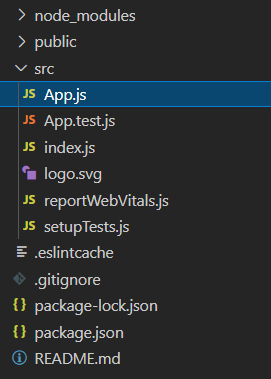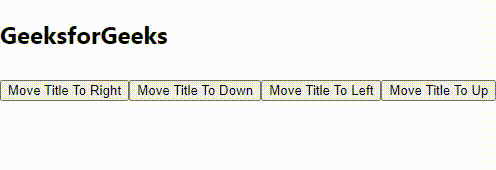# How to change the position of the element dynamically in ReactJS ?

• Last Updated : 22 Apr, 2021

In this article we will learn to change the position of an element dynamically using ReactJS

Approach: We are going to use following steps:

• Assume the position of our element is 0 on x-coordinate and 0 on y-coordinate.
• Then we will add/subtract some value from x/y coordinate depending on the direction we are moving.
• Keep all of these values in the state so that we can see the change in real-time
• Update the coordinates in state and we will see the position of element changing.

Setting up environment and Execution:

• Step 1: Create React App command

`npx create-react-app foldername`
• Step 2: After creating your project folder, i.e., folder name, move to it using the following command:

`cd foldername`

Project Structure: It will look like the following.## App.js

 `import React, { Component } from ``"react"``;`` ` `class App extends Component {`` ` `  ``// Create state``  ``state = {``    ``xoffset: 0,``    ``yoffset: 0,``    ``delta: 10,``  ``};``  ` `  ``moveTitleToDown = () => {``    ``this``.setState(``      ``{ yoffset: ``this``.state.yoffset ``        ``+ ``this``.state.delta });``  ``};``  ``moveTitleToRight = () => {``    ``this``.setState(``      ``{ xoffset: ``this``.state.xoffset ``        ``+ ``this``.state.delta });``  ``};``  ``moveTitleToLeft = () => {``    ``this``.setState(``      ``{ xoffset: ``this``.state.xoffset ``        ``- ``this``.state.delta });``  ``};``  ``moveTitleToUp = () => {``    ``this``.setState(``      ``{ yoffset: ``this``.state.yoffset ``        ``- ``this``.state.delta });``  ``};``  ` `  ``render() {``    ``return` `(``      ``
``        ``{``/* Element to Move Dynamically */``}``        ````          ``GeeksforGeeks``        ````  ` `        ``{``/* Move Controls */``}``        ``
``          ````          ````          ````          ````        ``
``      ``
``    ``);``  ``}``}``  ` `export ``default` `App;`

Step to Run Application: Run the application using the following command from the root directory of the project:

`npm start`

Output: Now open your browser and go to http://localhost:3000/, click on buttons to see outputMy Personal Notes arrow_drop_up